Скачать презентацию The Cost of Capital n Cost of capital

9171f6d5bb73b14b629c823b092f6256.ppt

• Количество слайдов: 37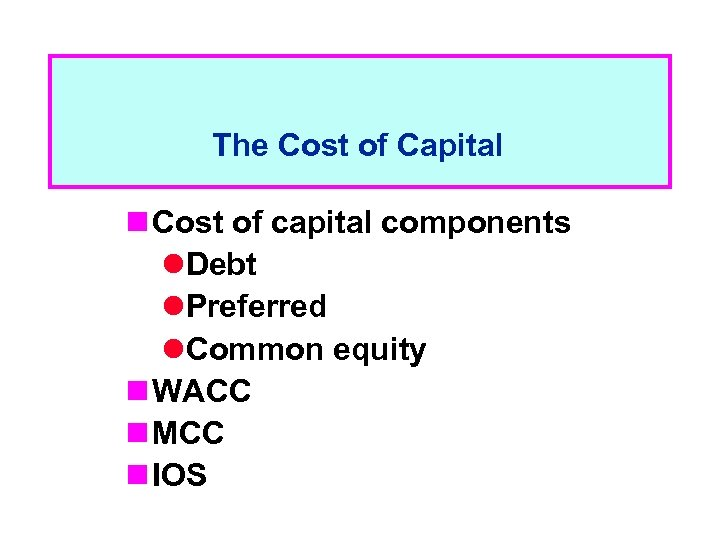The Cost of Capital n Cost of capital components l. Debt l. Preferred l. Common equity n WACC n MCC n IOSWhat sources of capital should be included in a firm’s WACC? n Long-term debt n Preferred stock n Common equity: l. Retained earnings l. New common stock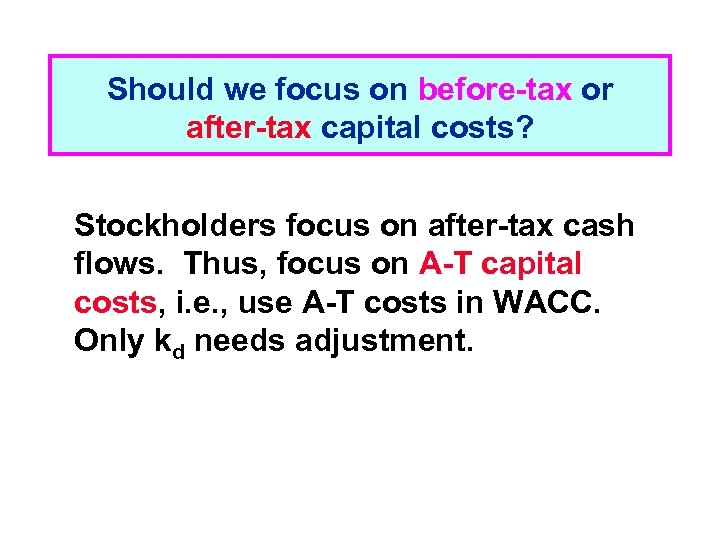Should we focus on before-tax or after-tax capital costs? Stockholders focus on after-tax cash flows. Thus, focus on A-T capital costs, i. e. , use A-T costs in WACC. Only kd needs adjustment.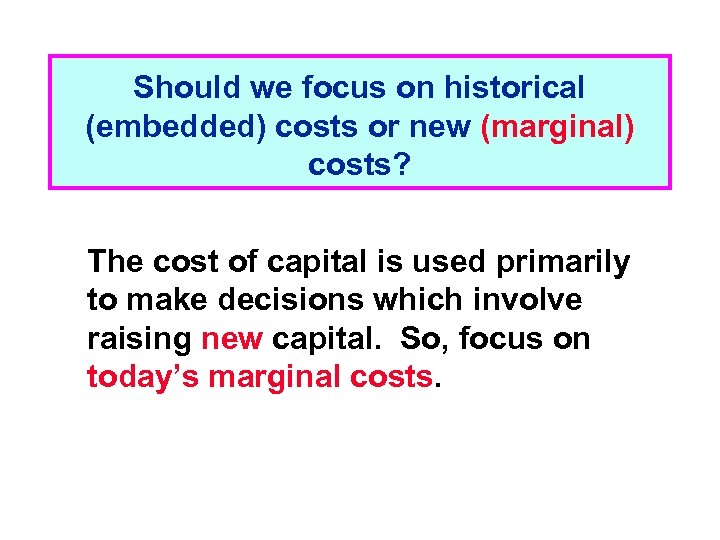Should we focus on historical (embedded) costs or new (marginal) costs? The cost of capital is used primarily to make decisions which involve raising new capital. So, focus on today’s marginal costs.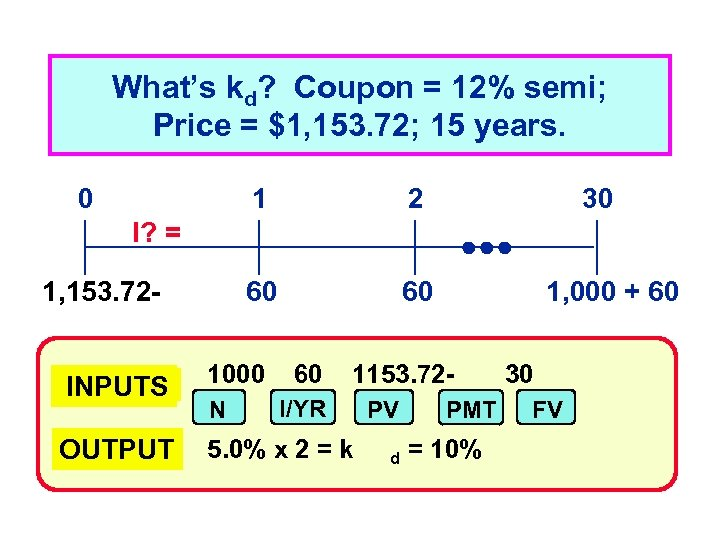What’s kd? Coupon = 12% semi; Price = \$1, 153. 72; 15 years. 0 1 2 60 30 60 I? = 1, 153. 72 INPUTS 1000 OUTPUT 5. 0% x 2 = k N 60 1, 000 + 60 1153. 72 - I/YR PV d PMT = 10% 30 FV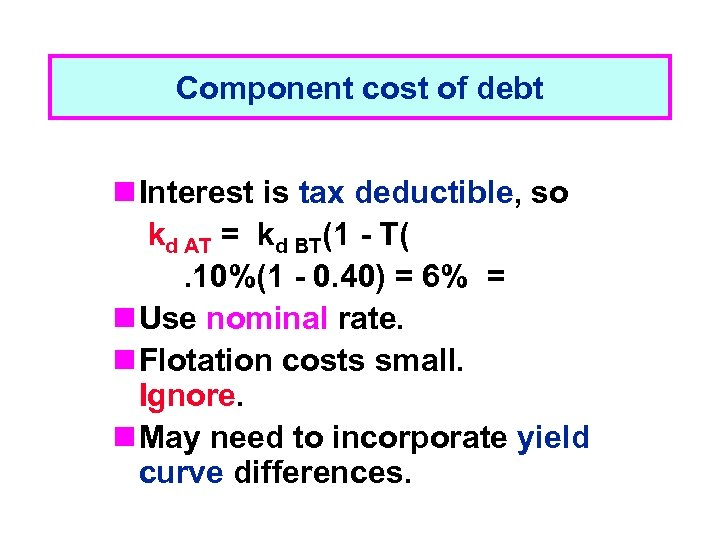Component cost of debt n Interest is tax deductible, so kd AT = kd BT(1 - T(. 10%(1 - 0. 40) = 6% = n Use nominal rate. n Flotation costs small. Ignore. n May need to incorporate yield curve differences.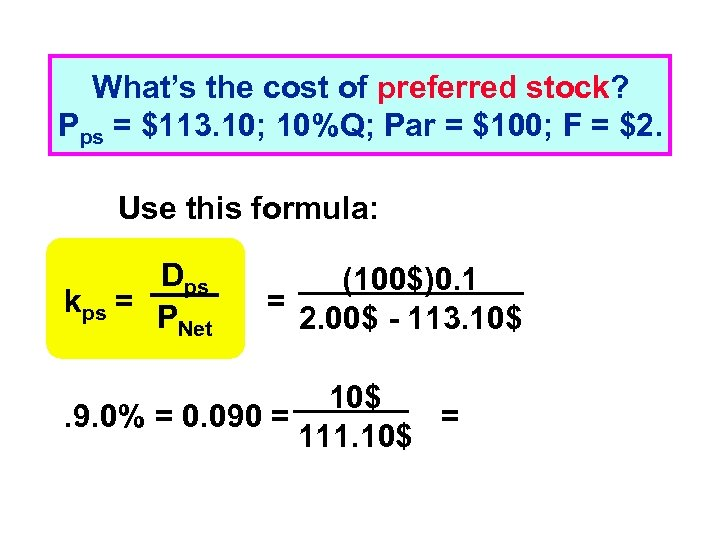What’s the cost of preferred stock? Pps = \$113. 10; 10%Q; Par = \$100; F = \$2. Use this formula: Dps kps = P Net (100\$)0. 1 = 2. 00\$ - 113. 10\$. 9. 0% = 0. 090 = = 111. 10\$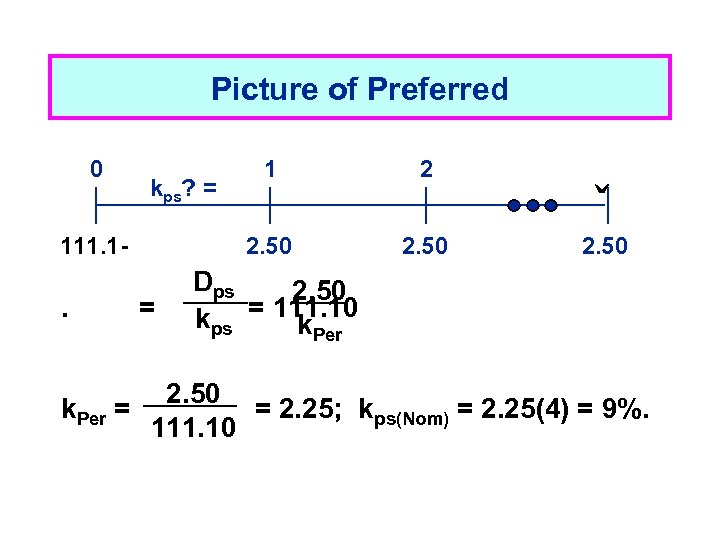Picture of Preferred 0 111. 1 - . = 2 ˬ 2. 50 kps? = 1 2. 50 Dps 2. 50 kps = 111. 10 k. Per 2. 50 k. Per = = 2. 25; kps(Nom) = 2. 25(4) = 9%. 111. 10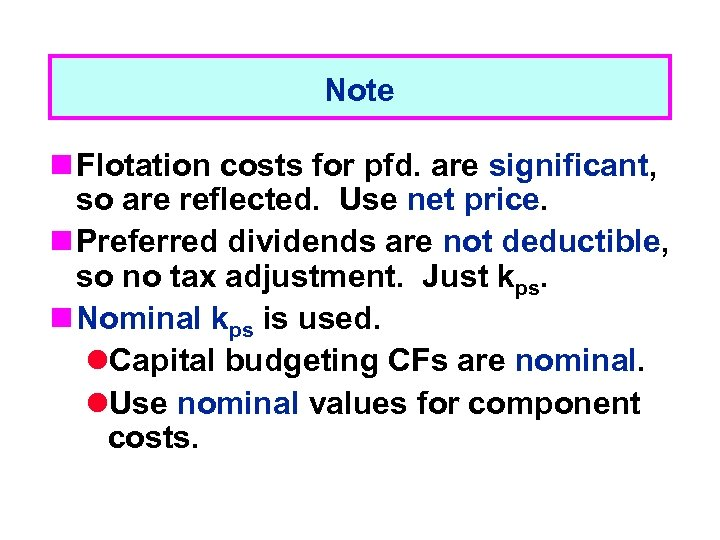Note n Flotation costs for pfd. are significant, so are reflected. Use net price. n Preferred dividends are not deductible, so no tax adjustment. Just kps. n Nominal kps is used. l. Capital budgeting CFs are nominal. l. Use nominal values for component costs.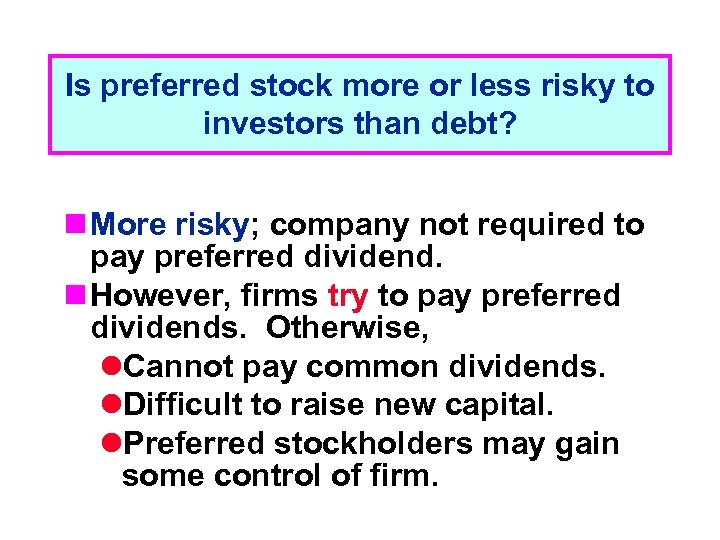Is preferred stock more or less risky to investors than debt? n More risky; company not required to pay preferred dividend. n However, firms try to pay preferred dividends. Otherwise, l. Cannot pay common dividends. l. Difficult to raise new capital. l. Preferred stockholders may gain some control of firm.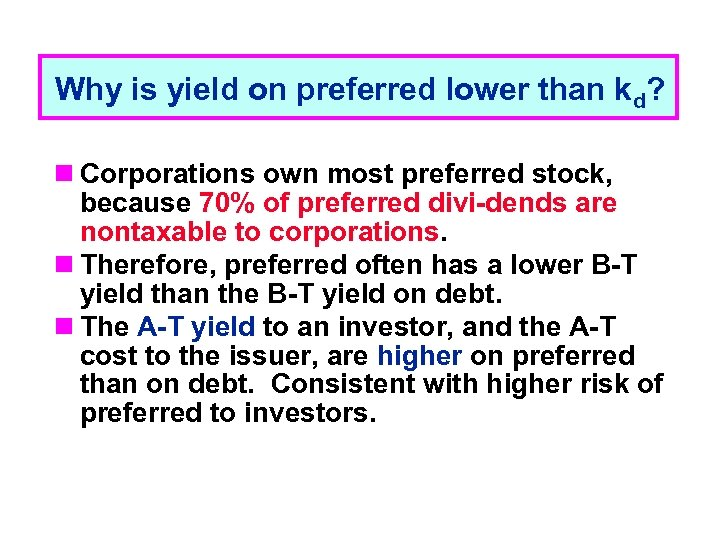Why is yield on preferred lower than kd? n Corporations own most preferred stock, because 70% of preferred divi-dends are nontaxable to corporations. n Therefore, preferred often has a lower B-T yield than the B-T yield on debt. n The A-T yield to an investor, and the A-T cost to the issuer, are higher on preferred than on debt. Consistent with higher risk of preferred to investors.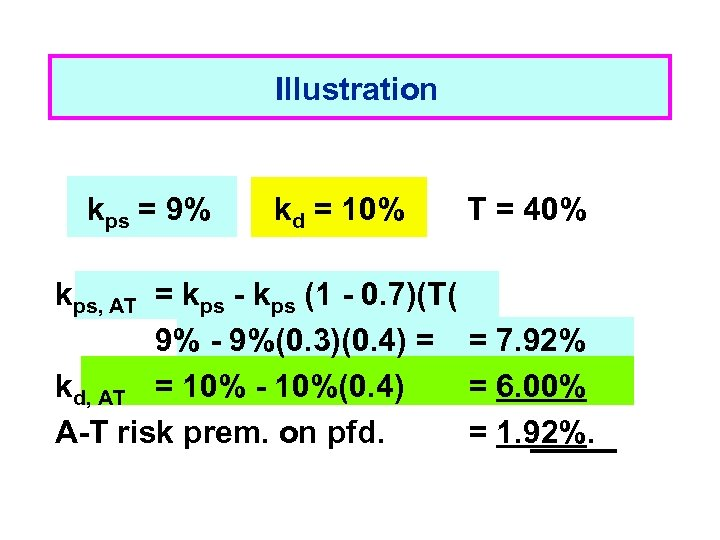Illustration kps = 9% kd = 10% T = 40% kps, AT = kps - kps (1 - 0. 7)(T( 9% - 9%(0. 3)(0. 4) = = 7. 92% kd, AT = 10% - 10%(0. 4) = 6. 00% A-T risk prem. on pfd. = 1. 92%.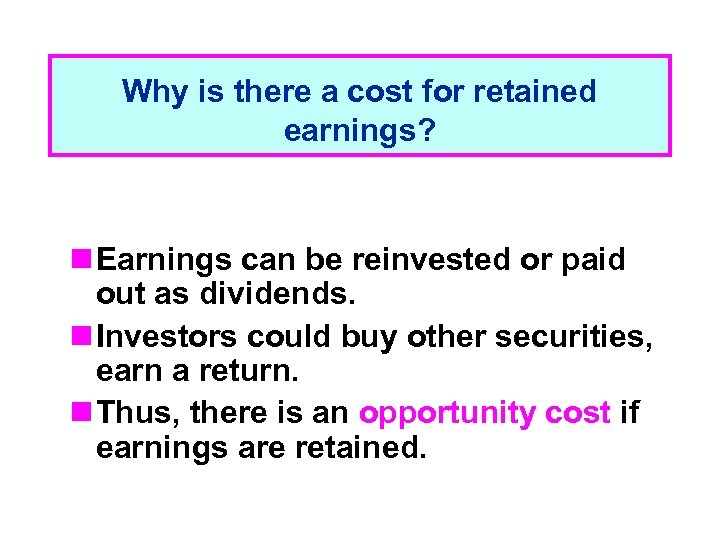Why is there a cost for retained earnings? n Earnings can be reinvested or paid out as dividends. n Investors could buy other securities, earn a return. n Thus, there is an opportunity cost if earnings are retained.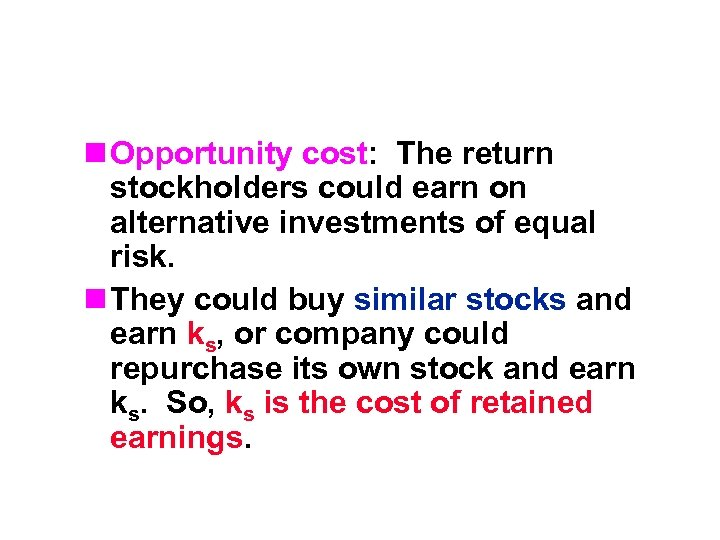n Opportunity cost: The return stockholders could earn on alternative investments of equal risk. n They could buy similar stocks and earn ks, or company could repurchase its own stock and earn ks. So, ks is the cost of retained earnings.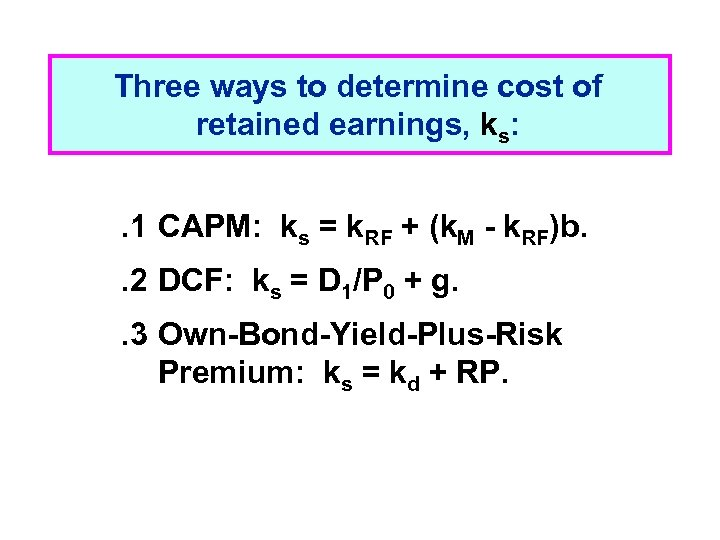Three ways to determine cost of retained earnings, ks: . 1 CAPM: ks = k. RF + (k. M - k. RF)b. . 2 DCF: ks = D 1/P 0 + g. . 3 Own-Bond-Yield-Plus-Risk Premium: ks = kd + RP.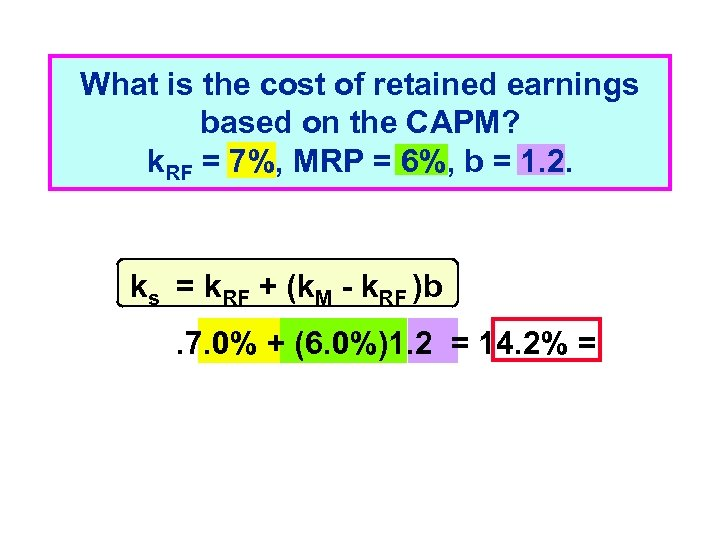What is the cost of retained earnings based on the CAPM? k. RF = 7%, MRP = 6%, b = 1. 2. ks = k. RF + (k. M - k. RF )b. 7. 0% + (6. 0%)1. 2 = 14. 2% =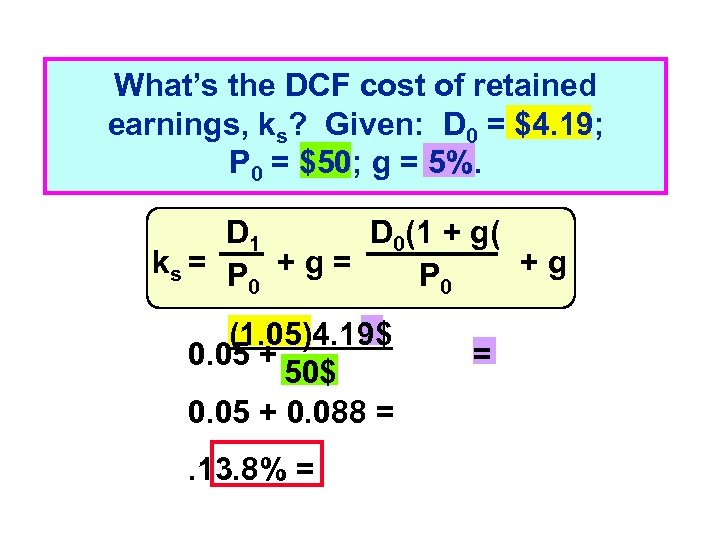What’s the DCF cost of retained earnings, ks? Given: D 0 = \$4. 19; P 0 = \$50; g = 5%. D 1 D 0(1 + g( ks = P + g = +g P 0 0 (1. 05)4. 19\$ 0. 05 + 50\$ 0. 05 + 0. 088 =. 13. 8% = =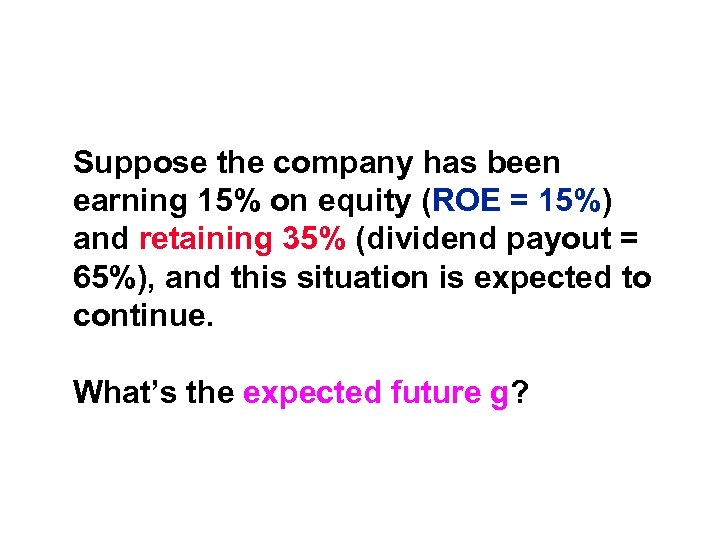Suppose the company has been earning 15% on equity (ROE = 15%) and retaining 35% (dividend payout = 65%), and this situation is expected to continue. What’s the expected future g?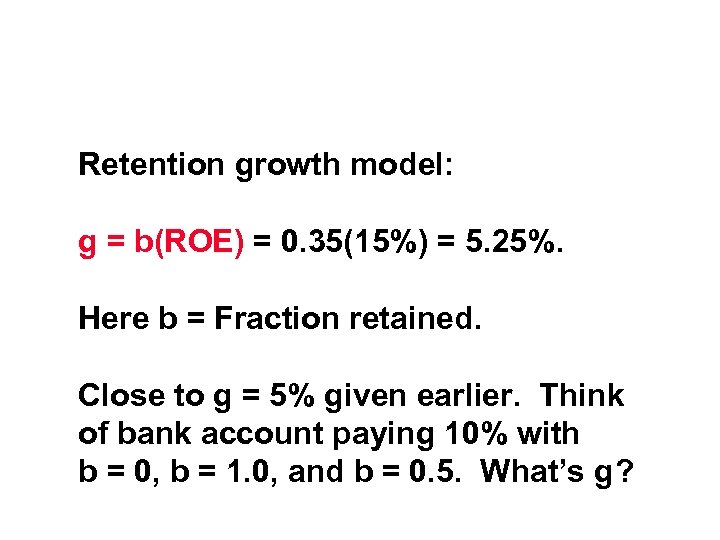Retention growth model: g = b(ROE) = 0. 35(15%) = 5. 25%. Here b = Fraction retained. Close to g = 5% given earlier. Think of bank account paying 10% with b = 0, b = 1. 0, and b = 0. 5. What’s g?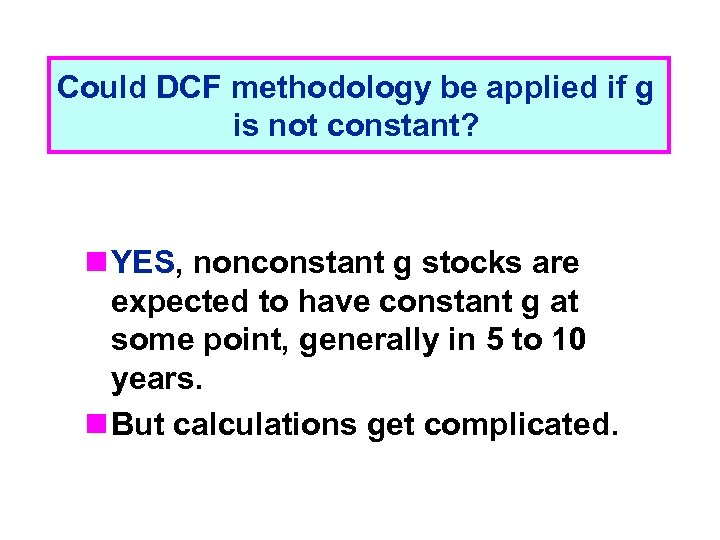Could DCF methodology be applied if g is not constant? n YES, nonconstant g stocks are expected to have constant g at some point, generally in 5 to 10 years. n But calculations get complicated.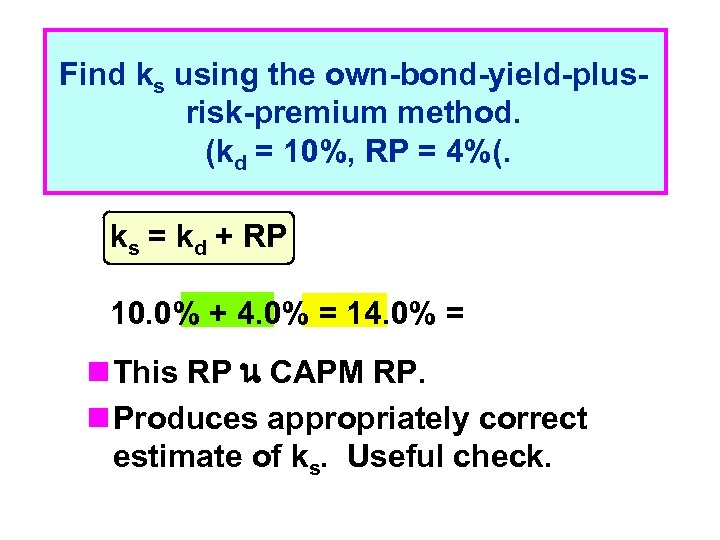Find ks using the own-bond-yield-plusrisk-premium method. (kd = 10%, RP = 4%(. ks = kd + RP 10. 0% + 4. 0% = 14. 0% = n This RP น CAPM RP. n Produces appropriately correct estimate of ks. Useful check.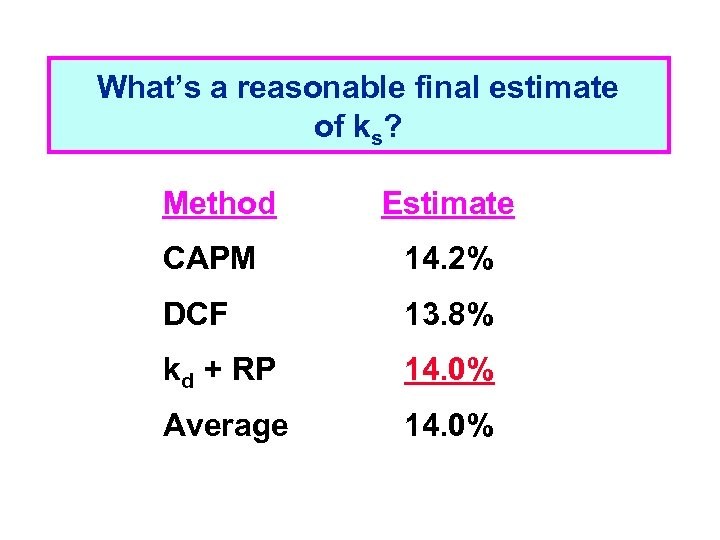What’s a reasonable final estimate of ks? Method Estimate CAPM 14. 2% DCF 13. 8% kd + RP 14. 0% Average 14. 0%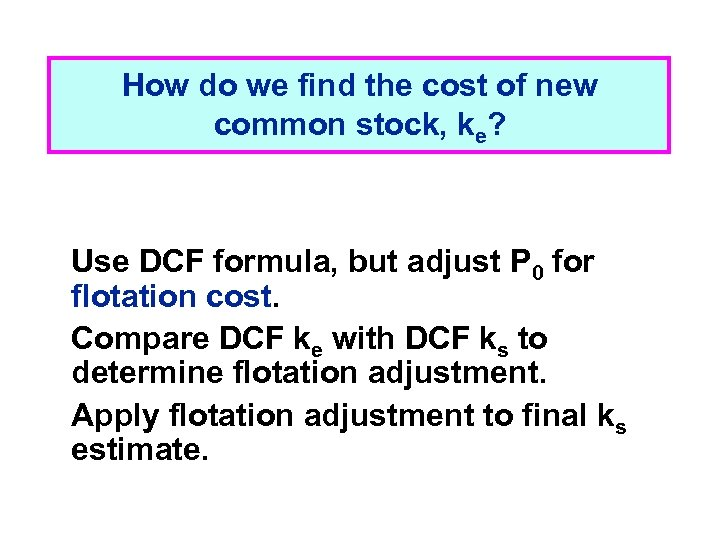How do we find the cost of new common stock, ke? Use DCF formula, but adjust P 0 for flotation cost. Compare DCF ke with DCF ks to determine flotation adjustment. Apply flotation adjustment to final ks estimate.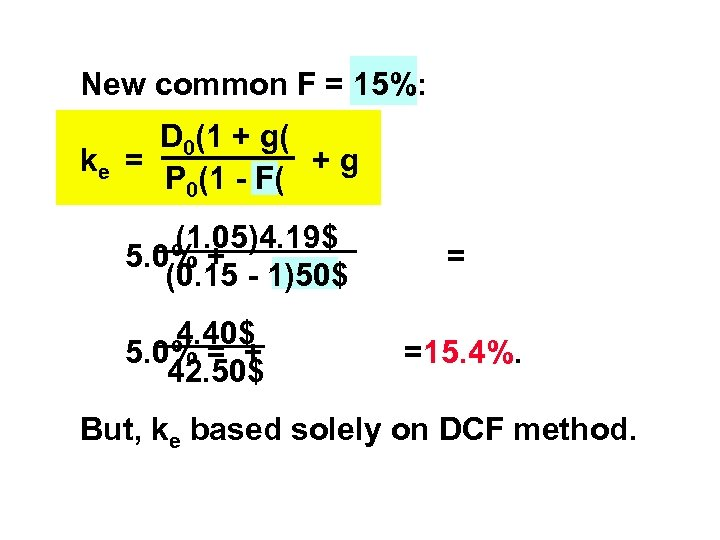New common F = 15%: D 0(1 + g( ke = +g P 0(1 - F( (1. 05)4. 19\$ 5. 0% + (0. 15 - 1)50\$ 4. 40\$ 5. 0% = + 42. 50\$ = =15. 4%. But, ke based solely on DCF method.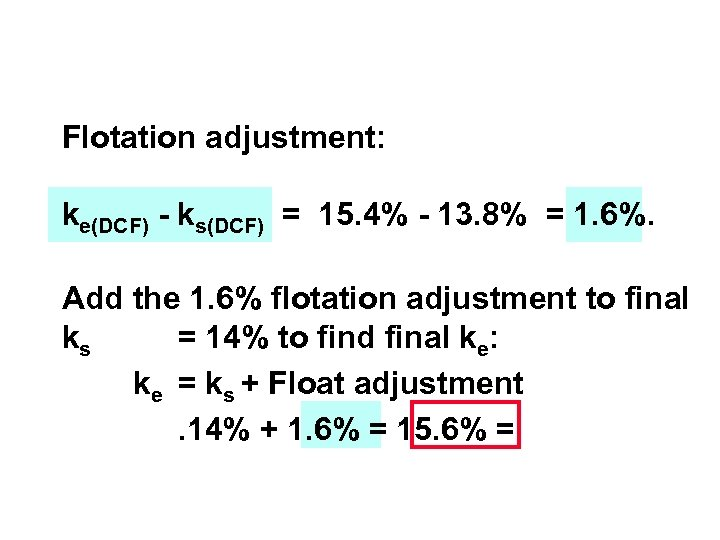Flotation adjustment: ke(DCF) - ks(DCF) = 15. 4% - 13. 8% = 1. 6%. Add the 1. 6% flotation adjustment to final ks = 14% to find final ke: ke = ks + Float adjustment. 14% + 1. 6% = 15. 6% =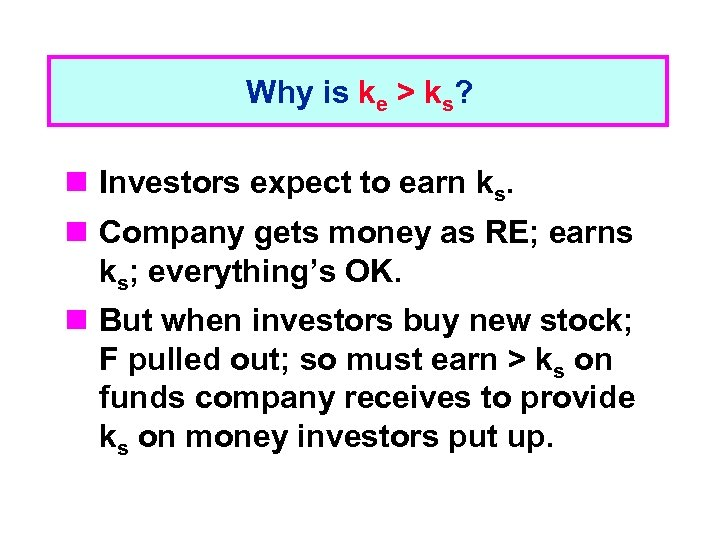Why is ke > ks? n Investors expect to earn ks. n Company gets money as RE; earns ks; everything’s OK. n But when investors buy new stock; F pulled out; so must earn > ks on funds company receives to provide ks on money investors put up.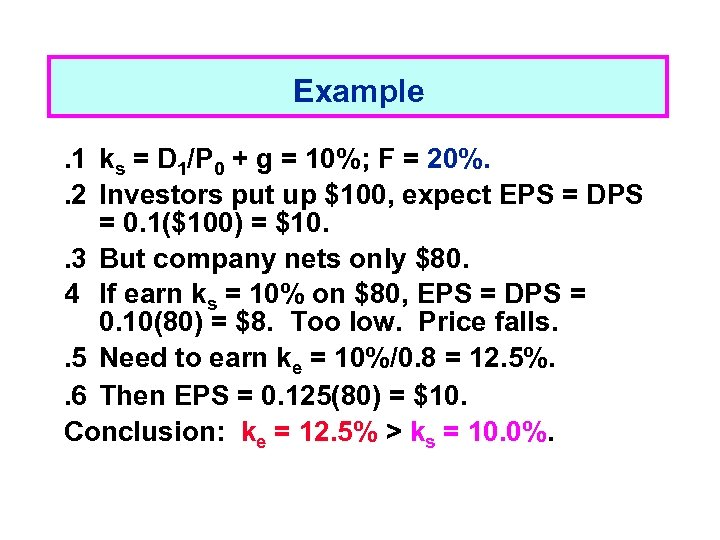Example. 1 ks = D 1/P 0 + g = 10%; F = 20%. . 2 Investors put up \$100, expect EPS = DPS = 0. 1(\$100) = \$10. . 3 But company nets only \$80. 4 If earn ks = 10% on \$80, EPS = DPS = 0. 10(80) = \$8. Too low. Price falls. . 5 Need to earn ke = 10%/0. 8 = 12. 5%. . 6 Then EPS = 0. 125(80) = \$10. Conclusion: ke = 12. 5% > ks = 10. 0%.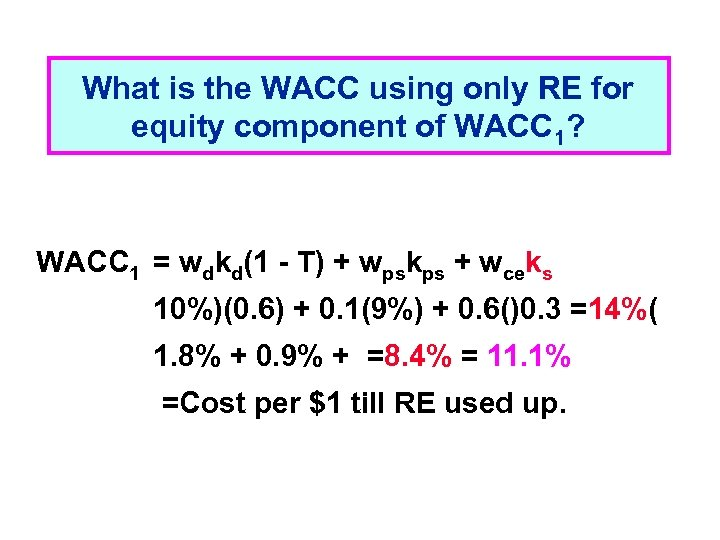What is the WACC using only RE for equity component of WACC 1? WACC 1 = wdkd(1 - T) + wpskps + wceks 10%)(0. 6) + 0. 1(9%) + 0. 6()0. 3 =14%( 1. 8% + 0. 9% + =8. 4% = 11. 1% =Cost per \$1 till RE used up.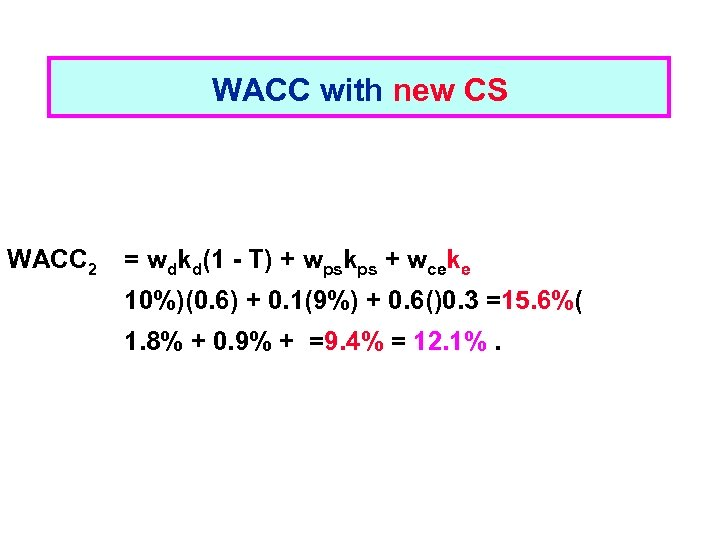WACC with new CS WACC 2 = wdkd(1 - T) + wpskps + wceke 10%)(0. 6) + 0. 1(9%) + 0. 6()0. 3 =15. 6%( 1. 8% + 0. 9% + =9. 4% = 12. 1%.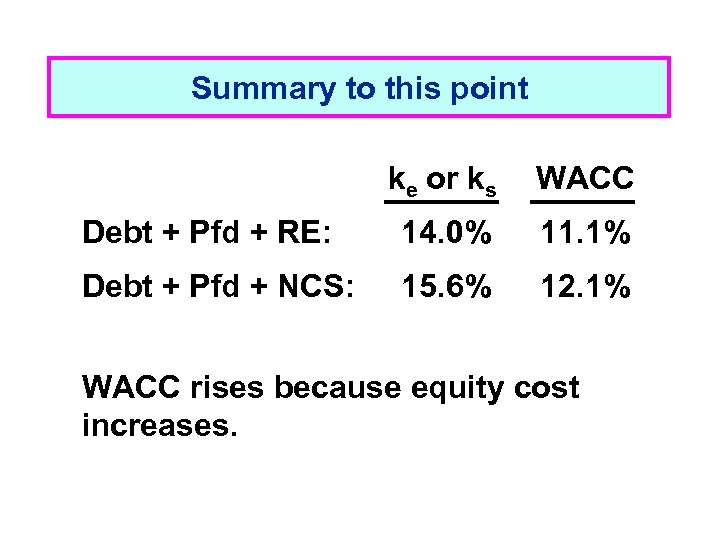Summary to this point ke or ks WACC Debt + Pfd + RE: 14. 0% 11. 1% Debt + Pfd + NCS: 15. 6% 12. 1% WACC rises because equity cost increases.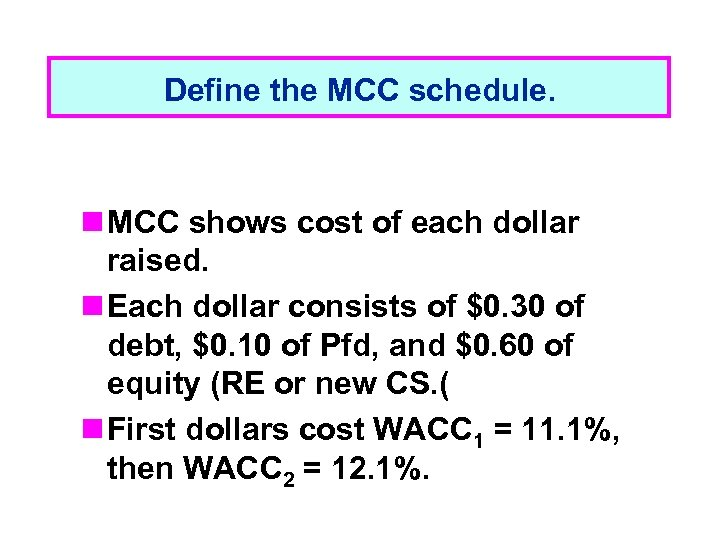Define the MCC schedule. n MCC shows cost of each dollar raised. n Each dollar consists of \$0. 30 of debt, \$0. 10 of Pfd, and \$0. 60 of equity (RE or new CS. ( n First dollars cost WACC 1 = 11. 1%, then WACC 2 = 12. 1%.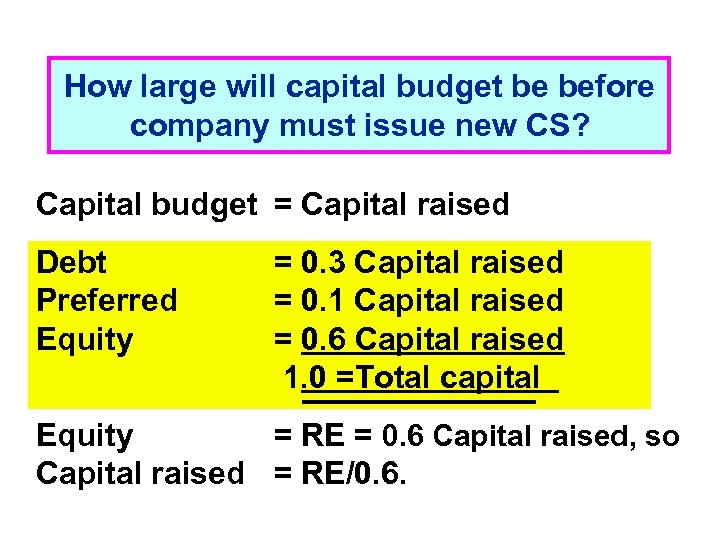How large will capital budget be before company must issue new CS? Capital budget = Capital raised Debt Preferred Equity = 0. 3 Capital raised = 0. 1 Capital raised = 0. 6 Capital raised 1. 0 =Total capital Equity = RE = 0. 6 Capital raised, so Capital raised = RE/0. 6.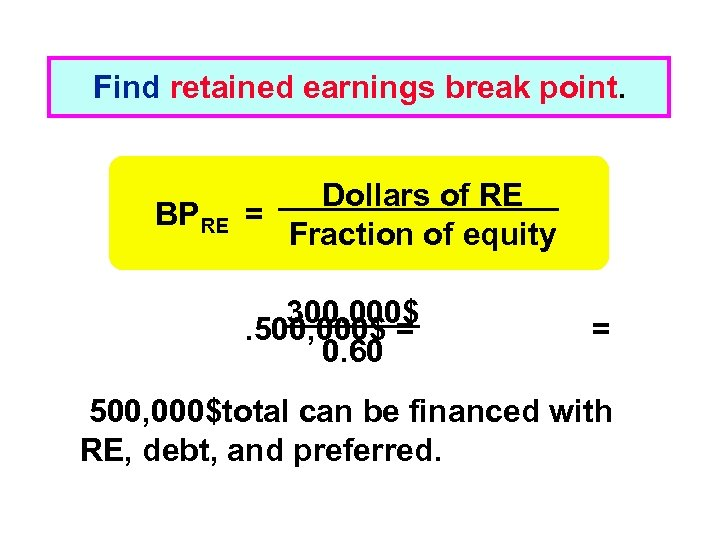Find retained earnings break point. BPRE Dollars of RE = Fraction of equity 300, 000\$. 500, 000\$ = 0. 60 = 500, 000\$total can be financed with RE, debt, and preferred.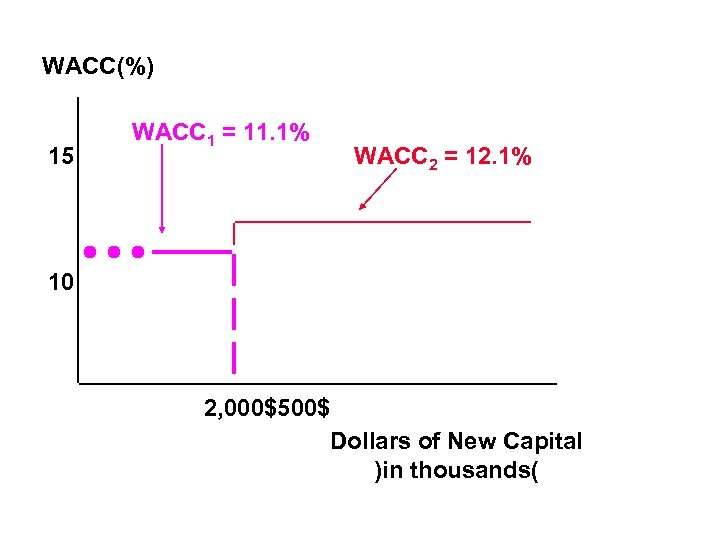WACC(%) 15 WACC 1 = 11. 1% WACC 2 = 12. 1% 10 2, 000\$500\$ Dollars of New Capital )in thousands(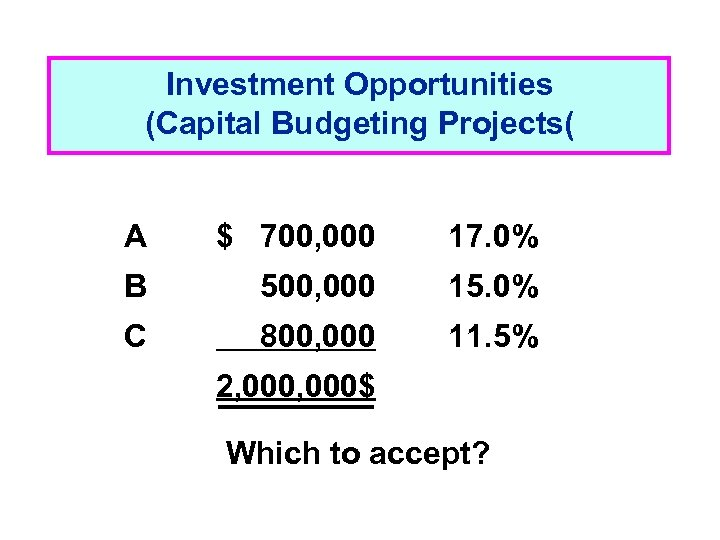Investment Opportunities (Capital Budgeting Projects( A \$ 700, 000 17. 0% B 500, 000 15. 0% C 800, 000 11. 5% 2, 000\$ Which to accept?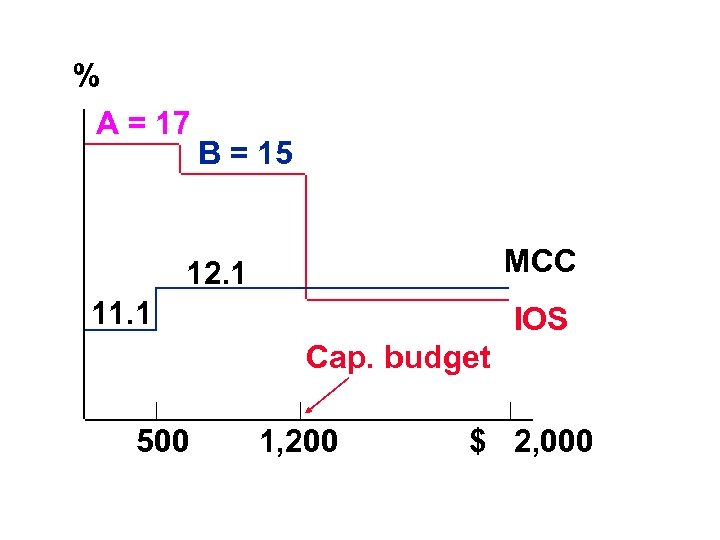% A = 17 B = 15 MCC 12. 1 11. 1 IOS Cap. budget 500 1, 200 \$ 2, 000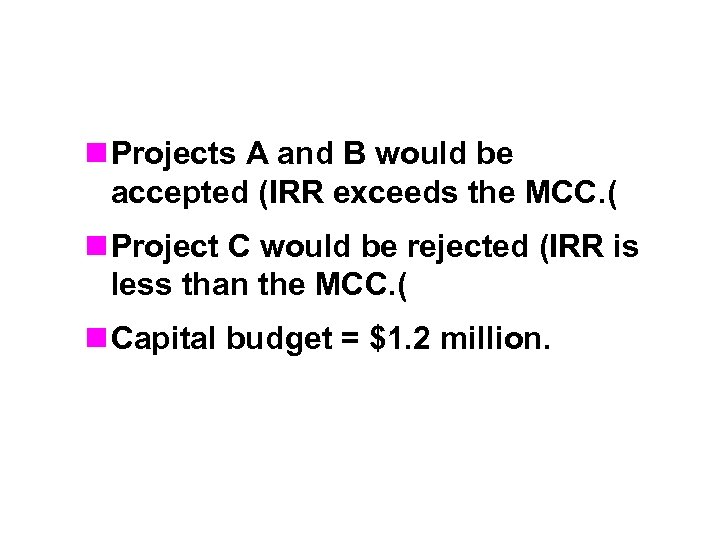n Projects A and B would be accepted (IRR exceeds the MCC. ( n Project C would be rejected (IRR is less than the MCC. ( n Capital budget = \$1. 2 million.